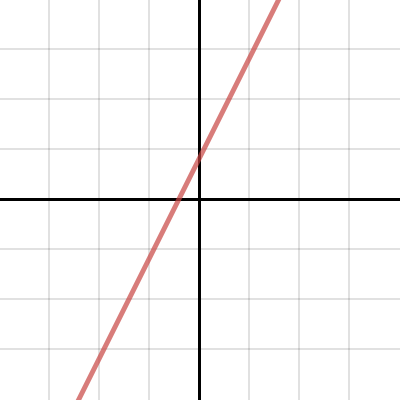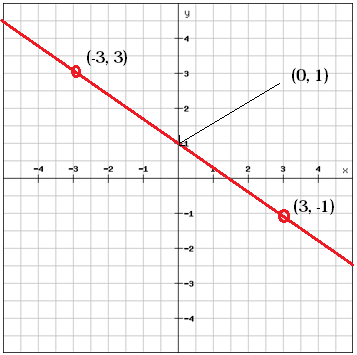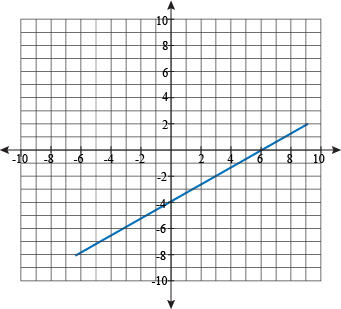## Pre-Algebra Tutorial

#### Intro

After understanding the where and how to find coordinates on a graph, we must understand the relationship between the points. This relationship is known as slope and will be a very popular term used for the rest of your math career. Slope is used in linear equations, meaning it is a straight line. Below is an example of a linear equation or line. This line is a positive relationship because it has a positive slope which will be explained below.The slope equation is written as: y=mx+b
This is known as slope intercept form
Seems confusing, but lets break it down: y and x are the coordinates, m is the slope, and b is the y-intercept. Now the y-intercept is just a fancy term used for “where does the line cross the y-axis.” So, b from the graph above is very close to 1 because that is where the line crosses the y-axis. To acquire the slope, we need to use this equation: m=(y2-y1)/(x2-x1). Yes, this is an equation inside of an equation. But don’t worry, we only have to solve for slope and we will have our answer. In this equation, we must pick any 2 points on the line (x1,y1) and (x2,y2). Try to pick points that have whole numbers to make this as easy as possible. For this example we will use points (0, 1) and (-2,-3) on the graph above. m=(-3-1)/(-2-0)= (-4)/(-2)= 2, m=2. Finally, the slope intercept form for this graph would be y=2x+1. If we decide to use x=3, we can solve for y: y=(2)(3)+1= 7. Therefore, when x=3, y=7 even though we cannot actually see it on the graph.

Example 1:The example already provides the slope intercept form of y=3x+25. m=3 and b=25 in this equation, meaning the slope is 3 and the y-intercept is 25. We need to prove the slope is correct by using the formula m=(y2-y1)/(x2-x1). So: m= (19-4)/(-2+7)= (15)/(5)= 3. Notice that -2+7 is the same as -2-(-7).

Example 2:It is important to understand that the graph for example 2 has a negative relationship. Example 2 provides the y-intercept (b=1) and 2 points that we can use to solve for the slope (m). m=(-1-3)/(3+3) =(-4)/(6) =-2/3. When the slope (m) is negative like in this example, the line will slant the opposite direction as a positive one as in example 1. The slope intercept form for this would be y=(-2/3)x+1

Example 3:
Sometimes we will be given information with no image or graph, and sometimes we have to scramble the equation to solve for the missing information (yikes). No problem; we already have the tools needed to solve for it.
Story time- Kim decides that she needs to plot points on a graph to solve the slope of her ramp for her skateboard. Unfortunately, she can’t find any graph paper and doesn’t have a steady hand to draw grid lines. She estimates that the ramp goes underground and will have the point of (-5,-8). She then estimates that the top of the ramp will have a point of (4,7). To acquire the slope intercept form we must first solve the slope and, second, find the y-intercept.
Slope: m=(-8-7)/(-5-4) =(-15)/(-9) =5/3. We have determined this is a positive slope
y-intercept (b): y=mx+b –> b=y-mx –> choose a point to plug into the equation: b=7-(5/3)(4) =1/3

…that’s a lot

#### Sample Problem

1. Using the graph below, state the y-intercept, solve the slope and provide the slope intercept form2. You are provided with the following information: the slope for a line is 3/4 and one point on the line is (0,-3). Can the slope intercept form be found? Can another point on the line be found? Explain.

3. You are given the points (-3,-5) and (-12,-10). Is this slope positive or negative? Explain. What is the slope?

#### Solution

1. y-intercept (b) is -4 since this is where the line crosses the y-axis. I will use the points (0,-4) and (6,0) to solve for slope since they are the easiest points for the equation: m=(0+4)/(6-0) =4/6 =2/3. Therefore, the slope intercept form is y=(2/3)x-4

2. Yes. The point given (0,-3) is actually the y-intercept. This means we have b=-3. We can then use the slope of m=3/4 in the equation: y=mx+b –> y=(3/4)x-3. To find another point, you can substitute x for any variable to solve for y. For example, if x=12 then y=(3/4)12-3 =9-3 =6 and the point will be (12,6).

3. Slope: m=(-10+5)/(-12+3) =5/9. Since this is a positive number, the slope will be positive.

# About The Author

 Zachary P Math, Financing, And Accounting I am a recent graduate from Kent State University with an Associate of Applied Business and a Bachelor of Business Administration. During my studies, I excelled at math, financing, and accounting and was offered tutoring jobs at the university. Unfortunately, I had to decline the jobs at the time du...
6 SubjectsKnowRo Tutor
8 Tutorials
Ashtabula, OH
Get Tutoring Info

^